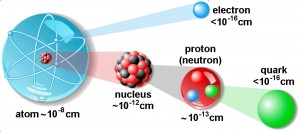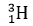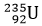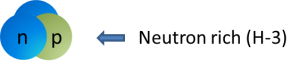# Module 1: Structure of Matter

### 1.1 Introduction

For the purpose of this course, we can use a simple model of matter. According to this model, the matter is made of substances and interactions. Substances are made of atoms.

The structure of atoms is presented below.Fig. 1-1 Structure of the Atoms

These are the four basic interactions:

1. Gravitational
2. Electromagnetic
3. Nuclear strong
4. Nuclear weak

### 1.1.1 Gravitational

The gravitational field is responsible for the attraction between masses of substance. Although the gravitational force carrier particle has yet to be discovered, the hypothetical particle is called the graviton. The gravitational interaction range is infinite.

### 1.1.2 Electromagnetic

The electromagnetic field is responsible for electric and magnetic interactions. The electromagnetic force carrier particle is called the photon and is, in fact, a quantum of light (or electromagnetic wave). X-rays and gamma rays are electromagnetic waves. The electromagnetic interaction range is also infinite.

### 1.1.3 Nuclear strong

The nuclear strong interaction is responsible for holding particles together inside the nucleus. The nucleus is composed of protons and neutrons. Protons have positive electric charge and neutrons are neutral (i.e. no net charge). Very large forces are necessary to hold together such a structure. The nuclear strong force carrier particle is called the gluon. The nuclear strong interaction has a range of 10-15 m (diameter of a proton).

### 1.1.4 Nuclear weak

The weak nuclear field is responsible for beta radioactivity (described later). The weak force carrier particles are called weak gauge bosons. The nuclear weak interaction has a range of 10-17 m (1% of the diameter of a proton).

### 1.2 The Atom

The fundamental structural blocks of substance are the atoms. More than 2000 different atoms have been identified. They are grouped in more than 105 different classes. These classes are assembled in a table called the “Periodic Table of the Elements”. Mendeleev discovered a periodicity of elements back in the 19th century. The periodicity is supported by the chemical properties of each element. These properties, in turn, stem from the arrangement of electrons around the nucleus.

The electrons are situated at comparatively large distances (compared to nuclear dimensions) around the nucleus. Electrons, which are much lighter than protons and neutrons, have a negative electric charge of 1.6 x 10-19 C. (C is the notation for electric charge unit called coulomb in “System International” – SI – unit system)
The electron mass is equal to 0.000549 amu, (one atomic mass unit – amu – is taken as 1/12 the mass of C-12 and is equal to 1.6604 x 10-27 kg).

Electrons move around the nucleus in shells, situated at different distances from the nucleus. Starting from the closest one, the shells are named K, L, M, N, … These shells are further divided into sub-shells called s, p, d, f, … Interactions between electrons and the nucleus are electromagnetic in nature. Therefore, the carrier particle is the photon (quantum of light). When electrons jump from one shell to another or outside of the atom, a quantum of light is emitted or absorbed by the atom.

When the number of electrons around the nucleus equals the number of protons inside the nucleus, the atom is electrically neutral. Different types of interactions can remove one or more electrons from a shell and thus create a positive ion. However, when the number of electrons is greater than the number of protons in the nucleus, we have a negative ion. The energy required to remove an electron from an atom is called the ionization energy. When radiation causes ionization of an atom, we call it ionizing radiation.

### 1.3 The Nucleus

The nucleus of an atom is composed of heavy particles called protons and neutrons.

A proton is a stable particle with a positive electric charge of 1.6 x 10-19 C, and a mass of 1.007276 amu.

A neutron is an electrically neutral particle with a mass of 1.008665 amu. Outside the nucleus, the neutron is unstable. It disintegrates into a proton, an electron, and an electron antineutrino.

The atomic number (Z) represents the total number of protons inside the nucleus. It is the same as the number of electrons surrounding the nucleus in neutral atoms. It is also called the order number, referring to the position in the periodic table. All atoms having the same atomic number have the same position in the periodic table. Different atoms having the same number of protons, but a different number of neutrons are called isotopes (“iso” for the same, “top” for position). In this case, the atomic number (Z) remains unchanged.

The number of neutrons is usually equal to or greater than the number of protons. The sum of the two is called the mass number (A) and gives the atomic weight of the atoms.

We recognize an atom by the name of the element (or atomic number) and by the mass number. For example,means that this atom is an isotope of Hydrogen (atomic number 1, and it occupies the first position in the periodic table) with a mass number (A) of 3. Therefore, inside the nucleus, there are one proton (Z=1) and two neutrons (A-Z, or 3-1 = 2) and around the nucleus is an electron.means that this atom has 92 protons (occupies the 92-nd position in the periodic table) and a mass number of 235. The number of neutrons is thus 143 (A-Z, or 235-92 = 143). The number of electrons is 92.

Although the SI notation for the naming of nuclides is the one shown above, in a day by day life, their notation is simplified. For example, instead ofwe simply use H-3, and instead of, we use U-235. In this course, we will adopt the simplified notation of nuclides.

Holding together two or more positively charged particles, with the exception of H-1 (which has one proton), can only be accomplished by a very strong interaction.

Due to the infinite range of the electromagnetic interaction, and to the short range of the strong force, neutrons are essential for the stability of the nucleus. For relatively small numbers of protons (at the beginning of the periodic table), an equal number of neutrons is sufficient to hold the nucleus together. As the atomic number increases, the number of neutrons required for stability also increases. Therefore, the atomic weight number (A) for stable atoms is more than double that of the atomic number (Z). For all atomic numbers below 92, there are one or more stable isotopes. They form a so-called “island of stability” for that particular atomic number. If the number of neutrons is greater than the required number for nuclear stability, the isotope is unstable and considered a neutron-rich nucleus. Conversely, if the number of neutrons is less than the required number for nuclear stability, that isotope is also unstable, but is now considered a proton-rich nucleus.Fig. 1-2 Types of Atoms

Unstable isotopes are called radioisotopes or radionuclides. They will keep disintegrating until they reach nuclear stability. This phenomenon is called radioactivity.